### Discover more content...

Enter some keywords in the search box above, we will do our best to offer you relevant results.

### We're sorry!

We couldn't find any results for your search. Please try again with another keywords.

## 一. 引子

$$h_\theta(x)=\frac{1}{1+e^{-\theta^Tx}}$$

\begin{align*} J(\theta)&=-(ylogh_\theta(x)+(1-y)log(1-h_\theta((x)))\\ &=-ylog\frac{1}{1+e^{-\theta^Tx}}-(1-y)log(1-\frac{1}{1+e^{-\theta^Tx}}) \end{align*}\\

$y=1$ 的时候：

$$cost_1(\theta^Tx^{(i)})=(-logh_\theta(x^{(i)}))$$

$y=0$ 的时候：

$$cost_0((\theta^Tx^{(i)})=((-log(1-h_\theta(x^{(i)})))$$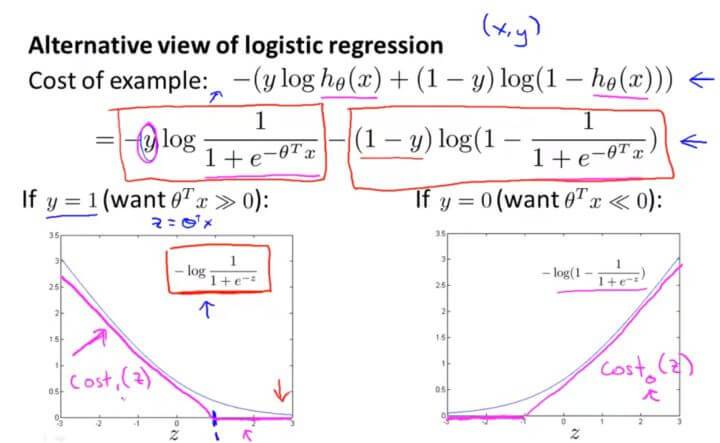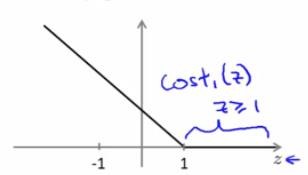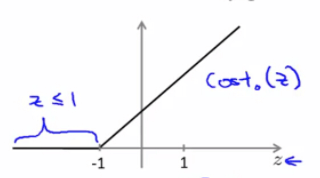$$J(\theta)=min_{\theta} \frac{1}{m}[\sum_{i=1}^{m}{y^{(i)}}(-logh_\theta(x^{(i)}))+(1-y^{(i)})((-log(1-h_\theta(x^{(i)})))]+\frac{\lambda}{2m}\sum_{j=1}^{n}{\theta_j^2}$$

$$min_{\theta} C[\sum_{i=1}^{m}{y^{(i)}}cost_1(\theta^Tx^{(i)})+(1-y^{(i)})cost_0(\theta^Tx^{(i)})]+\frac{1}{2}\sum_{j=1}^{n}{\theta_j^2}$$

$$h_{\theta}(x)=\left\{\begin{matrix} 1,\;\;if\; \theta^{T}x\geqslant 0\\ 0,\;\;otherwise \end{matrix}\right.$$

## 二. Large Margin Classification 大间距分类器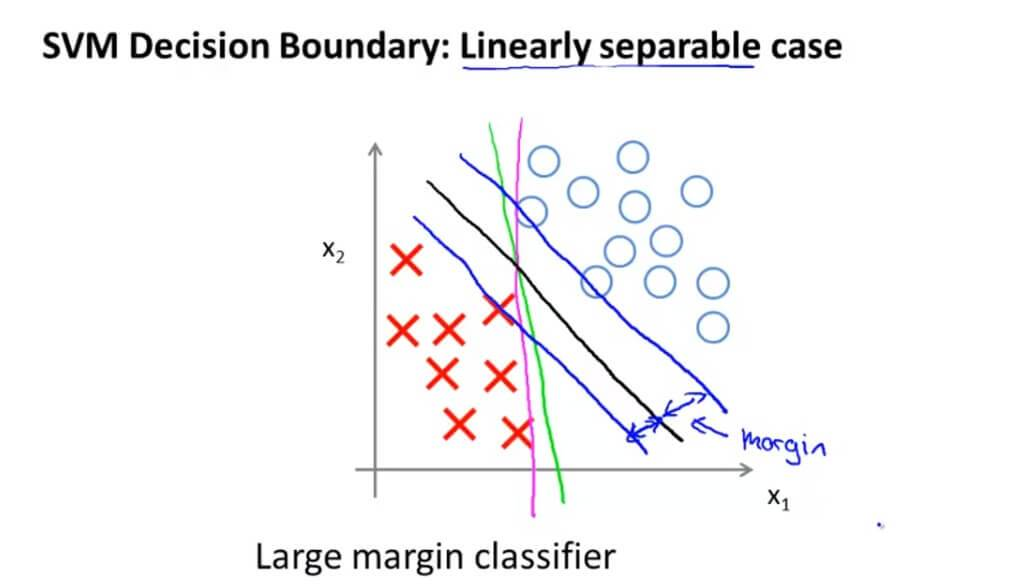$$min_{\theta} C[\sum_{i=1}^{m}{y^{(i)}}cost_1(\theta^Tx^{(i)})+(1-y^{(i)})cost_0(\theta^Tx^{(i)})]+\frac{1}{2}\sum_{j=1}^{n}{\theta_j^2}$$

$$min_{\theta}\frac{1}{2}\sum_{i=1}^{n}{\theta_j^2}\;\;\;\;\;\; \left\{\begin{matrix} \theta^Tx\geqslant 1,if \;y^{(i)}=1 \\ \theta^Tx\leqslant 1 ,if \;y^{(i)}=0 \end{matrix}\right.$$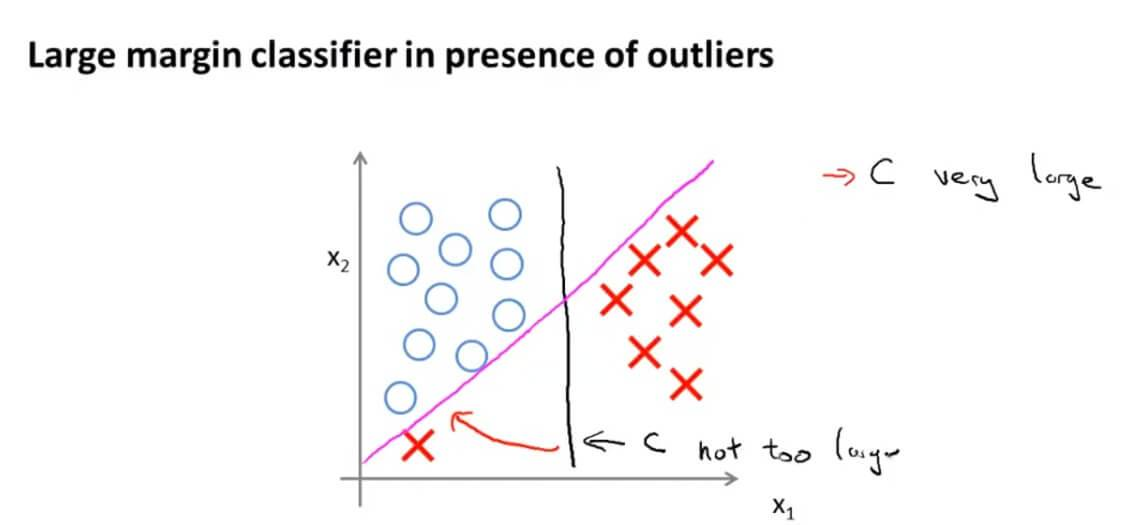### 推导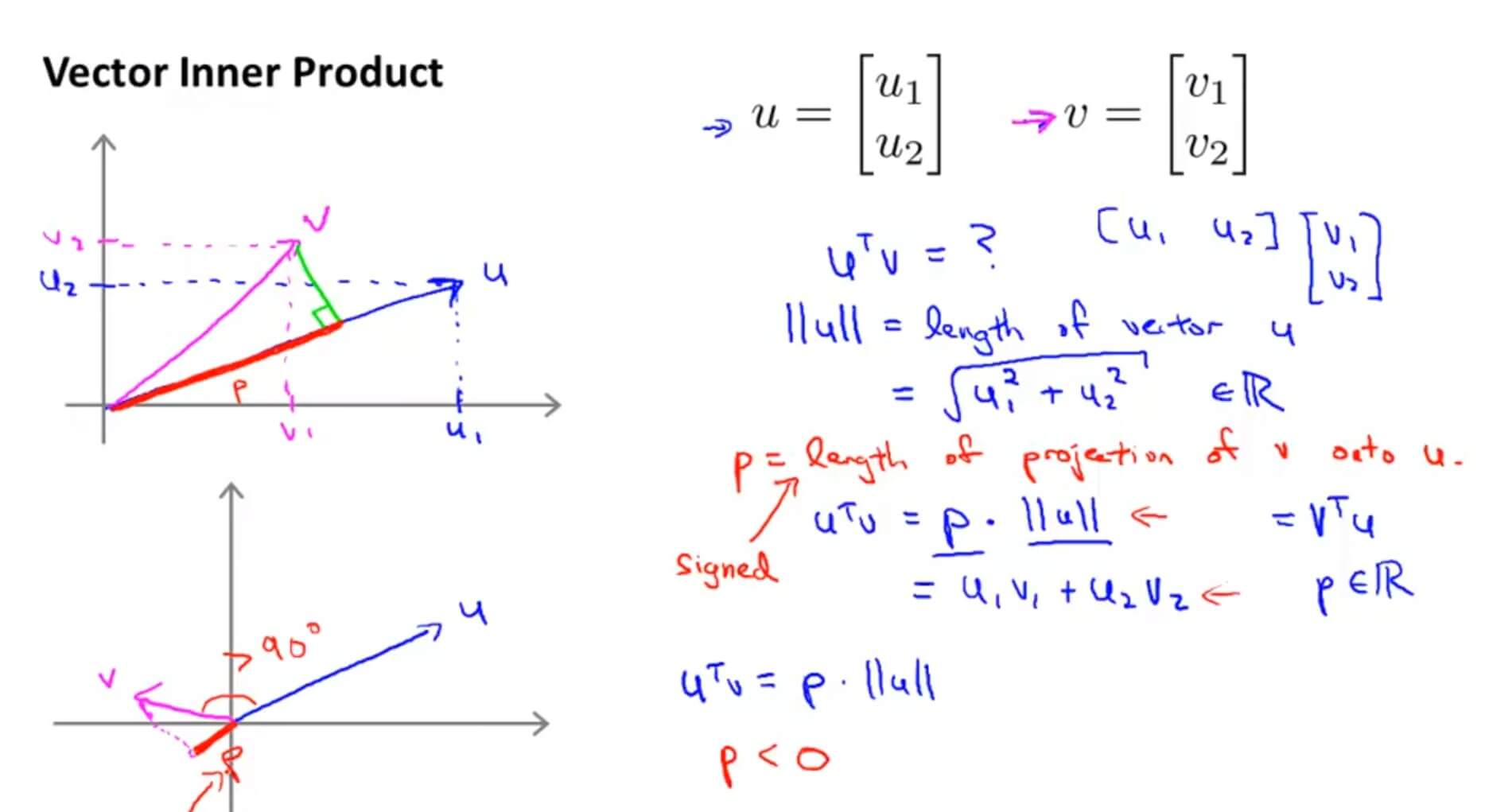$\left \| u \right \|$为 $\overrightarrow{u}$ 的范数，也就是向量 $\overrightarrow{u}$ 的欧几里得长度。

$$min_{\theta}\frac{1}{2}\sum_{i=1}^{n}{\theta_j^2}=\frac{1}{2}(\theta_1^2+\theta_2^2)=\frac{1}{2}(\sqrt{\theta_1^2+\theta_2^2})^2=\frac{1}{2}\left \| \theta \right \|^2$$

$$\left \| u \right \| = \sqrt{u_{1}^{2} + u_{2}^{2}}$$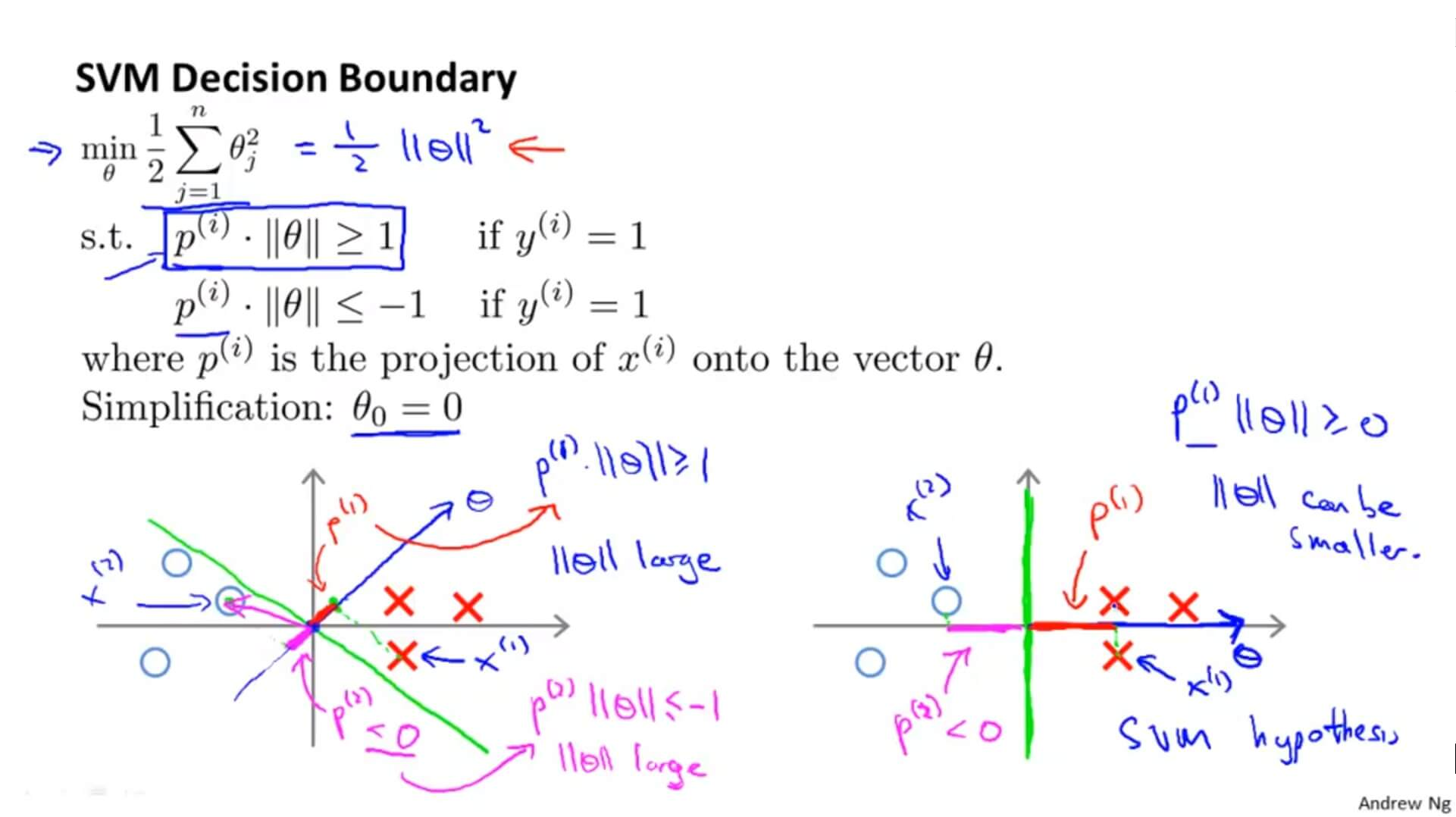(因为只有最大间距才能使 $p^{(i)}$ 大，从而 $||\theta||^2$ 值小)

## 三. Kernels

### 1. 定义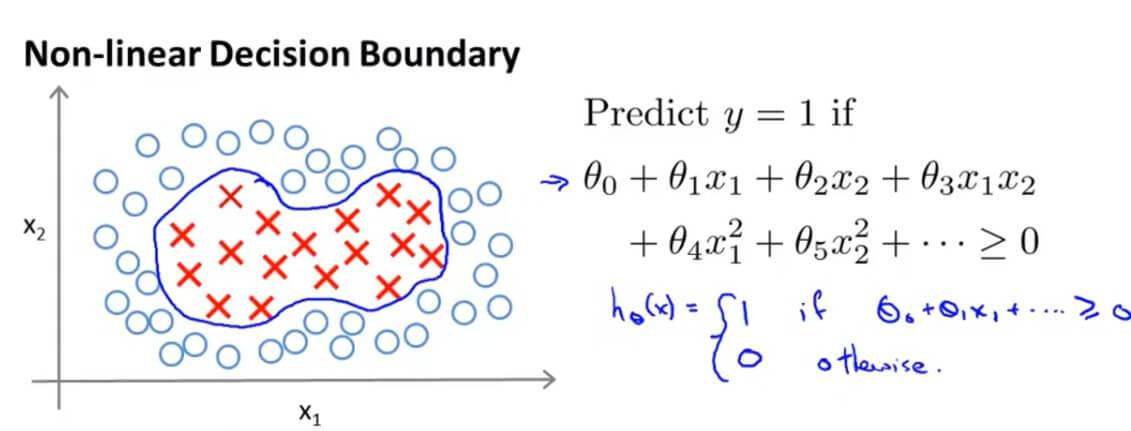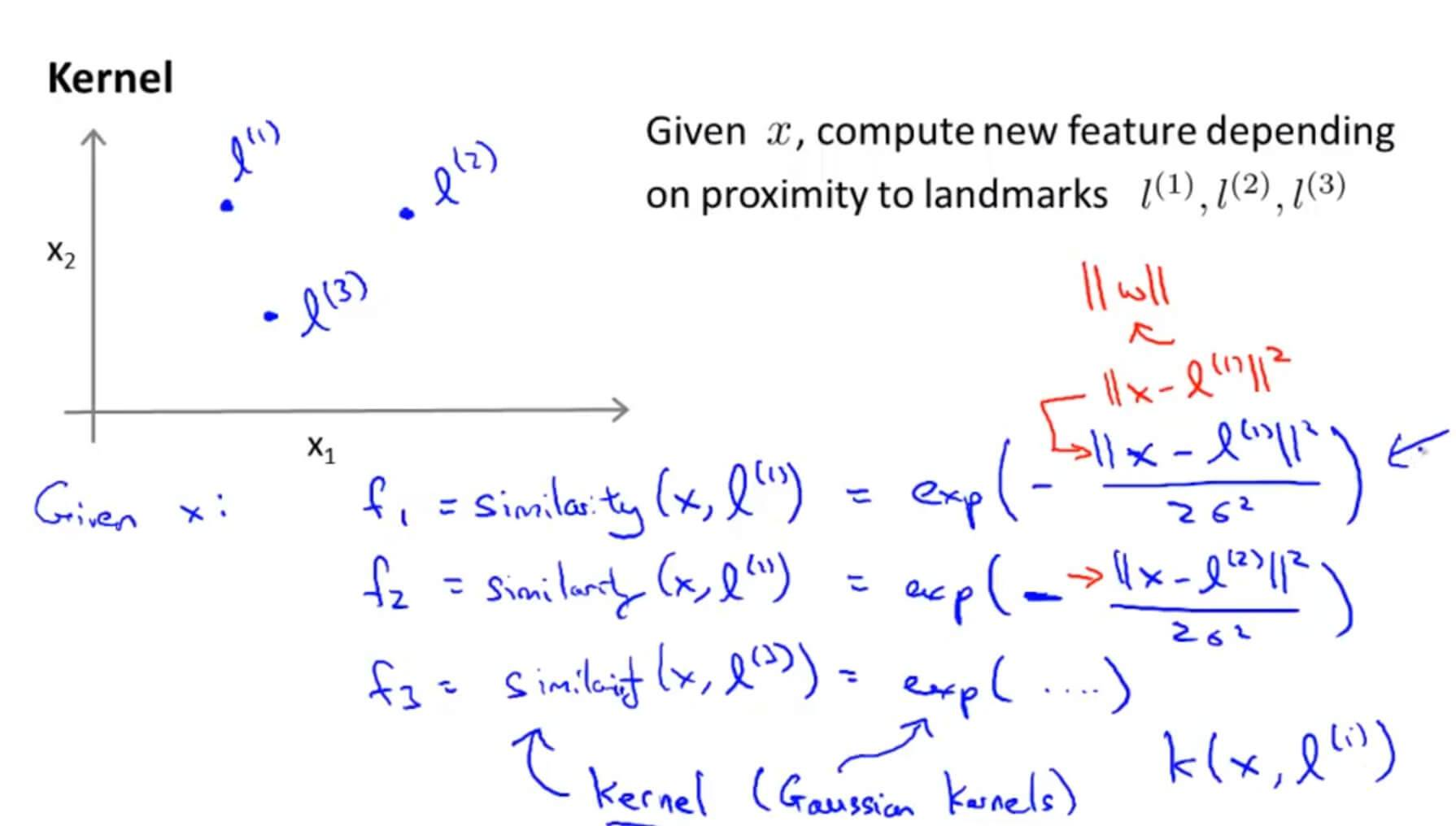$f_3=similarity(x,l^{(3)})=exp(-\frac{||x-l^{(3)}||^2}{2\sigma^2})$ 。这个表达式我们称之为核函数（Kernels），在这里我们选用的核函数是高斯核函数（Gaussian Kernels）。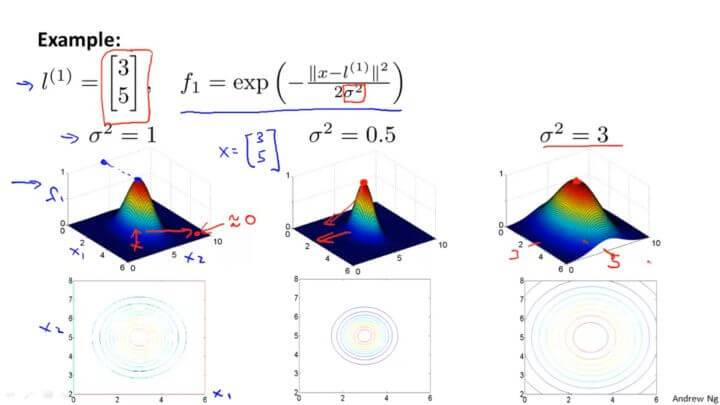### 2. 标记点选取

$$(x^{(1)},y^{(1)}),(x^{(2)},y^{(2)}),(x^{(3)},y^{(3)})\cdots(x^{(m)},y^{(m)})$$

$$l^{(1)}=x^{(1)},l^{(2)}=x^{(2)},l^{(3)}=x^{(3)}\cdots l^{(m)}=x^{(m)}$$

$$\begin{matrix} f^{(i)}_1=sim(x^{(i)},l^{(1)})\\ f^{(i)}_2=sim(x^{(i)},l^{(2)})\\ \vdots \\ f^{(i)}_m=sim(x^{(i)},l^{(3)})\\ \end{matrix}$$

$$f = \begin{bmatrix} f_0\\ f_1\\ f_2\\ \vdots \\ f_m \end{bmatrix}$$

$$min_{\theta} C[\sum_{i=1}^{m}{y^{(i)}}cost_1(\theta^Tf^{(i)})+(1-y^{(i)})cost_0(\theta^Tf^{(i)})]+\frac{1}{2}\sum_{j=1}^{n}{\theta_j^2}$$

## 四. SVMs in Practice

### 1. 使用流行库

• 参数 C
• 核函数（Kernel）

• 当特征维度 n 较高，而样本规模 m 较小时，不宜使用核函数，否则容易引起过拟合。

• 当特征维度 n 较低，而样本规模 m 足够大时，考虑使用高斯核函数。不过在使用高斯核函数前，需要进行特征缩放（feature scaling）。

• 当核函数的参数 $\sigma^2$ 较大时，特征 $f_i$ 较为平缓，即各个样本的特征差异变小，此时会造成欠拟合（高偏差，低方差），如下图上边的图，

• 当 $\sigma^2$ 较小时，特征 $f_i$ 曲线变化剧烈，即各个样本的特征差异变大，此时会造成过拟合（低偏差，高方差），如下图下边的图：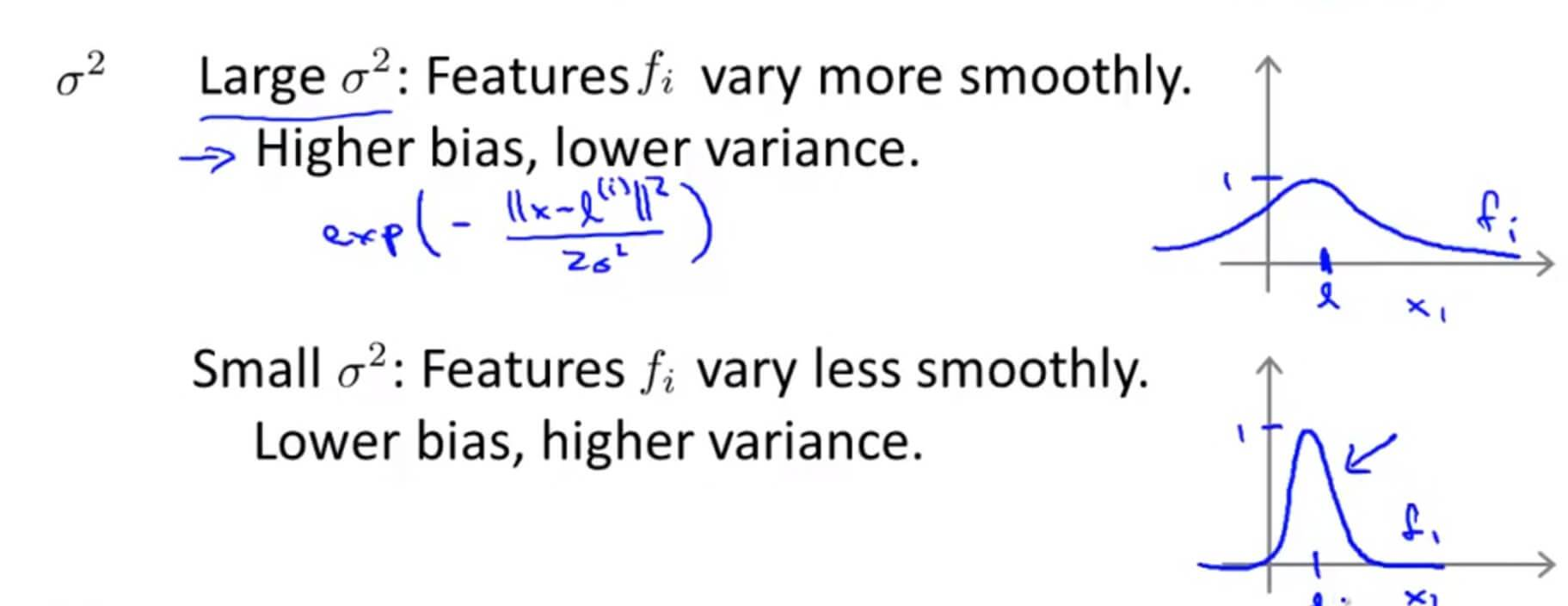### 2. 多分类问题

1. 轮流选中某一类型 i ，将其视为正样本，即 “1” 分类，剩下样本都看做是负样本，即 “0” 分类。
2. 训练 SVM 得到参数 $\theta^{(1)},\theta^{(2)},\cdots,\theta^{(K)}$ ，即总共获得了 K−1 个决策边界。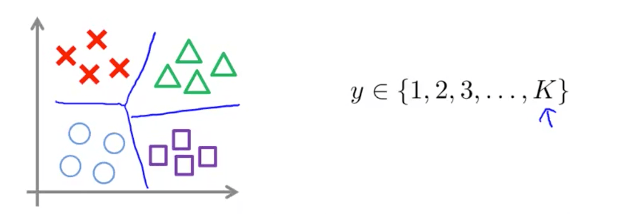（1）逻辑回归；
（2）神经网络；
（3）SVM

## 四. Support_Vector_Machines 测试

### 1. Question 1

Suppose you have trained an SVM classifier with a Gaussian kernel, and it learned the following decision boundary on the training set:When you measure the SVM's performance on a cross validation set, it does poorly. Should you try increasing or decreasing C? Increasing or decreasing $\sigma^{2}$?

A. It would be reasonable to try decreasing C. It would also be reasonable to try increasing $\sigma^{2}$.

B. It would be reasonable to try increasing C. It would also be reasonable to try increasing $\sigma^{2}$.

C. It would be reasonable to try increasing C. It would also be reasonable to try decreasing $\sigma^{2}$.

D. It would be reasonable to try decreasing C. It would also be reasonable to try decreasing $\sigma^{2}$.

### 2. Question 2

The formula for the Gaussian kernel is given by similarity $(x,l^{(1)})=exp(-\frac{\left \| x-l^{(1)} \right \|^{2}}{2\sigma^{2} })$ .

The figure below shows a plot of f1=similarity $(x,l^{(1)})$ when $\sigma^{2} = 1$.Which of the following is a plot of f1 when $\sigma^{2} = 0.25$?

A.B.C.D.$\sigma^{2}$ 变小图像变瘦高。

### 3. Question 3

The SVM solves

$$min_{\theta} C \sum^{m}_{i=1}y^{(i)}cost_{1}(\theta^{T}x^{(i)})+(1-y^{(i)})cost_{0}(\theta^{T}x^{(i)})+\sum^{n}_{j=1}\theta^{2}_{j}$$

where the functions $cost_0(z)$ and $cost_1(z)$ look like this:

The first term in the objective is:

$$C \sum^{m}_{i=1}y^{(i)}cost_{1}(\theta^{T} x^{(i)})+(1-y^{(i)})cost_{0}(\theta^{T}x^{(i)})$$

This first term will be zero if two of the following four conditions hold true. Which are the two conditions that would guarantee that this term equals zero?

A. For every example with $y^{(i)}=0$, we have that $\theta^{T}x(i) \leqslant 0$.

B. For every example with $y^{(i)}=1$, we have that $\theta^{T}x(i) \geqslant 0$.

C. For every example with $y^{(i)}=0$, we have that $\theta^{T}x(i)\leqslant-1$.

D. For every example with $y^{(i)}=1$, we have that $\theta^{T}x(i)\geqslant 1$.

### 4. Question 4

Suppose you have a dataset with n = 10 features and m = 5000 examples.

After training your logistic regression classifier with gradient descent, you find that it has underfit the training set and does not achieve the desired performance on the training or cross validation sets.

Which of the following might be promising steps to take? Check all that apply.

A. Try using a neural network with a large number of hidden units.

B. Create / add new polynomial features.

C. Reduce the number of examples in the training set.

D. Use a different optimization method since using gradient descent to train logistic regression might result in a local minimum.

A.增多神经网络的隐藏层可以解决欠拟合问题。
B.增加特征量可以解决欠拟合问题。
C.减少训练集样本，不行。
D.不是梯度下降函数到达最低值是代价函数。

### 5. Question 5

Which of the following statements are true? Check all that apply.

A. Suppose you have 2D input examples (ie, $x^{(i)} \in \mathbb{R}^2$). The decision boundary of the SVM (with the linear kernel) is a straight line.

B. If the data are linearly separable, an SVM using a linear kernel will return the same parameters $\theta$ regardless of the chosen value of C (i.e., the resulting value of $\theta$ does not depend on C).

C. If you are training multi-class SVMs with the one-vs-all method, it is not possible to use a kernel.

D. The maximum value of the Gaussian kernel (i.e., $sim(x,l^{(1)})$) is 1.

A. 线性是一条直线。
B. $min_{\theta} C[\sum_{i=1}^{m}{y^{(i)}}cost_1(\theta^Tx^{(i)})+(1-y^{(i)})cost_0(\theta^Tx^{(i)})]+\frac{1}{2}\sum_{j=1}^{n}{\theta_j^2}$， $\theta$正是由 C 的大小决定的。
C. 解决多分类问题可以用 SVM 。
D. 高斯核函数范围：[0,1]。

GitHub Repo：Halfrost-Field

Follow: halfrost · GitHubPrevious Post

Next Post